Checkout JEE MAINS 2022 Question Paper Analysis : Checkout JEE MAINS 2022 Question Paper Analysis :

# Kirchhoff's Second Law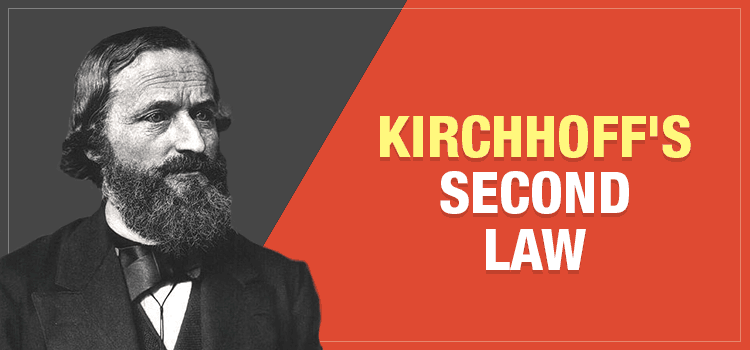Gustav Robert Kirchhoff, a German physicist, contributed to the fundamental understanding of electrical circuits. Kirchhoff’s Law or circuit Laws is composed of two mathematical equality equations that deal with resistance, current, and voltage in the lumped element model of electrical circuits. The laws are fundamental to circuit theory. They quantify how current flows and voltages vary through a loop in a circuit.

## What are Kirchhoff’s Laws?

There are two laws as follows:

• Kirchhoff’s second law, also known as Kirchhoff’s voltage law (KVL) states that the sum of all voltages around a closed loop in any circuit must be equal to zero. This again is a consequence of charge conservation and also conservation of energy.

Here in this short piece of article, we will be discussing Kirchhoff’s second law.

## Kirchhoff’s Voltage Law

Kirchhoff’s Second Law or the voltage law states that

The net electromotive force around a closed circuit loop is equal to the sum of potential drops around the loop

It is termed Kirchhoff’s Loop Rule, which is an outcome of an electrostatic field that is conservative.
Hence,

• If a charge moves around a closed loop in a circuit, it must gain as much energy as it loses.
• The above can be summarized as the gain in energy by the charge = corresponding losses through resistances
• Mathematically, the total voltage in a closed loop of a circuit is expressed as
$$\begin{array}{l}\sum V=0\end{array}$$
.

The below figure illustrates that the total voltage around a closed loop must be zero.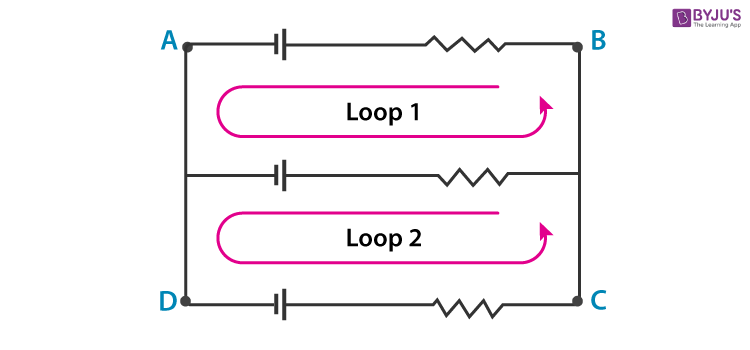This law manages the voltage drops at different branches in an electrical circuit. Consider one point on a closed loop in an electrical circuit. If somebody goes to another point in a similar ring, they will find that the potential at that second aspect might be not quite the same as the first point.

If they keep on setting off to some unique point on the loop, they may locate some extraordinary potential in that new area. If they go on further along that closed loop, eventually, they achieve the underlying point from where the voyage was begun.

That implies that they return to a similar potential point in the wake of the intersection through various voltage levels. It can be then again said that the gain in electrical energy by the charge is equal to corresponding losses in energy through resistances.

Related Article:

### Solving Circuit Using Kirchhoff’s Second Law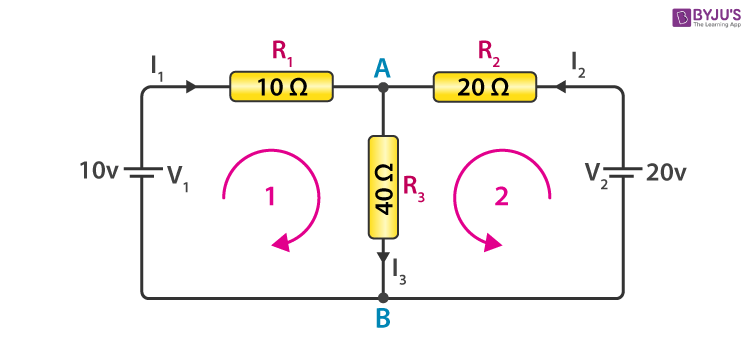Step 1: The first and foremost step is to draw a closed loop to a circuit. Once done with it, draw the direction of the flow of current.

Step 2: Defining our sign convention is very important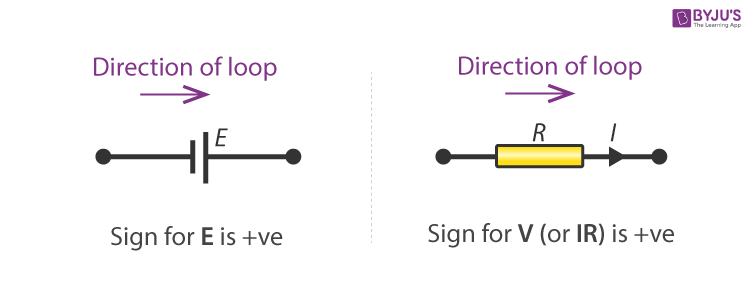Step 3: Using Kirchhoff’s first law, at B and A we get, I1+I2=I3

Step 4: By making use of the above convention and Kirchhoff’s Second Law,

From Loop 1 we have:

$$\begin{array}{l}10=R_{1}*I_{1}+R_{3}*I_{3}\end{array}$$

$$\begin{array}{l}=10I_{1}+40I_{3}\end{array}$$

$$\begin{array}{l}1=I_{1}+4I_{3}\end{array}$$

From Loop 2 we have :

$$\begin{array}{l}20=R_{2}*I_{2}+R_{3}*I_{3}\end{array}$$

$$\begin{array}{l}20I_{2}+40I_{3}\end{array}$$

$$\begin{array}{l}1=I_{2}+2I_{3}\end{array}$$

From Loop 3 we have :

$$\begin{array}{l}10-20=10I_{1}-20I_{2}\end{array}$$

$$\begin{array}{l}1=-I_{1}+2I_{2}\end{array}$$

By making use of Kirchhoff’s First law, I1+I2=I3

Equation reduces as follows (from Loop 1 ) :

$$\begin{array}{l}1=5I_{1}+4I_{2}\end{array}$$

Equation reduces as follows ( from Loop 2 ) :

$$\begin{array}{l}1=2I_{1}+3I_{2}\end{array}$$

This results in the following Equation:

$$\begin{array}{l}I_{1}=-\frac{1}{3}I_{2}\end{array}$$

From last three equations we get,

$$\begin{array}{l}1=\frac{1}{3}I_{2}+2I_{2}\end{array}$$

$$\begin{array}{l}I_{2}=0.429A\end{array}$$

$$\begin{array}{l}I_{1}=0.143A\end{array}$$

$$\begin{array}{l}I_{3}=0.286A\end{array}$$

### Advantages and Limitations of Kirchhoff’s Law

The advantages of the laws are:

• It calculates unknown voltages and currents easy
• The analysis and simplification of complex closed-loop circuits becomes manageable

Kirchhoff’s laws work under the assumption that there are no fluctuating magnetic fields in the closed loop. Electric fields and electromotive force could be induced, which results in the breaking of Kirchhoff’s rule under the influence of the varying magnetic field.

## Frequently Asked Questions – FAQs

### What is the other name of Kirchhoff’s first law?

Kirchhoff’s first law is also known as Kirchhoff’s current law (KCL).

### What is the other name of Kirchhoff’s second law?

Kirchhoff’s second law is also known as Kirchhoff’s voltage law (KVL).

### What is Kirchhoff’s second law?

Kirchhoff’s Second Law or the voltage law states that the net electromotive force around a closed circuit loop is equal to the sum of potential drops around the loop.

### What is Kirchhoff’s first law?

Kirchhoff’s first law, also known as Kirchhoff’s current law (KCL) states that the current flowing into a node must be equal to the current flowing out of the node.

### What are the Advantages of Kirchhoff’s Law?

It makes the calculation of unknown voltages and currents easy.

## Watch the video and learn basic electrical circuit concepts and learn to solve problems on Electrical Circuit, KCL, and KVL.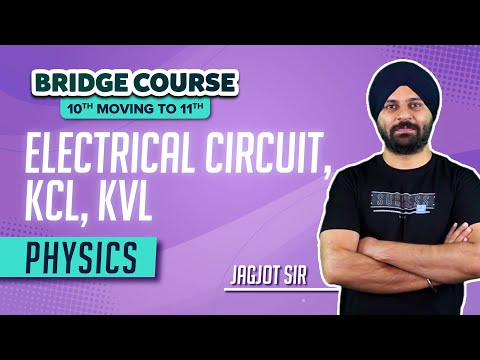Stay tuned with BYJU’S for more such interesting articles. Also, register to “BYJU’S – The Learning App” for loads of interactive, engaging Physics-related videos and unlimited academic assistance.

Test your knowledge on Kirchhoff's second law